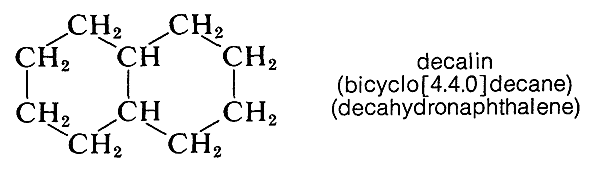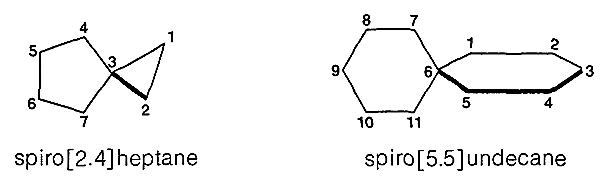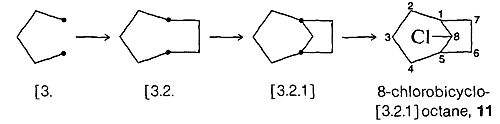# 12.8: Nomenclature of Polycycloalkanes

$$\newcommand{\vecs}{\overset { \rightharpoonup} {\mathbf{#1}} }$$ $$\newcommand{\vecd}{\overset{-\!-\!\rightharpoonup}{\vphantom{a}\smash {#1}}}$$$$\newcommand{\id}{\mathrm{id}}$$ $$\newcommand{\Span}{\mathrm{span}}$$ $$\newcommand{\kernel}{\mathrm{null}\,}$$ $$\newcommand{\range}{\mathrm{range}\,}$$ $$\newcommand{\RealPart}{\mathrm{Re}}$$ $$\newcommand{\ImaginaryPart}{\mathrm{Im}}$$ $$\newcommand{\Argument}{\mathrm{Arg}}$$ $$\newcommand{\norm}{\| #1 \|}$$ $$\newcommand{\inner}{\langle #1, #2 \rangle}$$ $$\newcommand{\Span}{\mathrm{span}}$$ $$\newcommand{\id}{\mathrm{id}}$$ $$\newcommand{\Span}{\mathrm{span}}$$ $$\newcommand{\kernel}{\mathrm{null}\,}$$ $$\newcommand{\range}{\mathrm{range}\,}$$ $$\newcommand{\RealPart}{\mathrm{Re}}$$ $$\newcommand{\ImaginaryPart}{\mathrm{Im}}$$ $$\newcommand{\Argument}{\mathrm{Arg}}$$ $$\newcommand{\norm}{\| #1 \|}$$ $$\newcommand{\inner}{\langle #1, #2 \rangle}$$ $$\newcommand{\Span}{\mathrm{span}}$$

There are many hydrocarbons and hydrocarbon derivatives with two or more rings having common carbon atoms. Such a substance is decalin, which has ten carbons arranged in two six-membered rings:Compounds of this type usually are named by attaching the prefix bicyclo to the name of the open-chain hydrocarbon with the same total number of carbon atoms as in the rings. Thus decalin, which has ten carbons in the ring system, is a bicyclodecane. Next, we have to have a way to specify the sizes of the rings, which is done by counting the number of carbon atoms in each of the chains connecting the two atoms that constitute the ring junctions or bridgeheads. Decalin has four carbons in each of two chains and none in the third. Therefore, decalin is bicyclo[4.4.0]decane. Notice that the numbers are enclosed in square brackets after the prefix “bicyclo” and before the name of the hydrocarbon. The numbers are listed in order of decreasing magnitude and are properly separated by periods, not commas. Some other examples follow:To name substituted polycycloalkanes, a numbering system is required. In the IUPAC system the main ring is the one containing the largest number of carbon atoms. Two of the carbons in the main ring serve as junctions for the main bridge, which is chosen to be as large as possible, consistent with the choice of the main ring. Additional rules are required for more complex cases, but these are not of interest to us here.

In numbering bicyclic ring systems that have two ring junctions, one of them is chosen as $$\ce{C_1}$$. The numbering proceeds along the longest chain of carbons to the next junction, then continues along the next longest chain, and finally is completed along the shortest chain. For example,Here, the main ring has seven carbons ($$\ce{C_1}$$ to $$\ce{C_7}$$) and there is a one-carbon bridge $$\left( \ce{C_8} \right)$$.

When the hydrocarbon rings have only one carbon in common, they are called spiranes and are given systematic names in accord with the following examples:Notice that for spiranes the numbering starts next to the junction point in the smaller ring.

The naming of tricycloalkanes follows the same general system.$$^7$$ The largest ring and its main linkage form a bicyclic system, and the location of the fourth or secondary linkage is shown by superscripts. The systematic name of the interesting hydrocarbon adamantane is given below as an example; its conformation also is shown. The largest ring in adamantane is eight-membered and the carbons that constitute it could be selected in several different ways. The carbon chosen as $$\ce{C_9}$$ lies between $$\ce{C_1}$$ and $$\ce{C_5}$$, not between the higher-numbered $$\ce{C_3}$$ and $$\ce{C_7}$$:To generate a structure from a name such as 8-chlorobicyclo[3.2.1]octane, $$11$$, start with a pair of junction atoms, connect them as prescribed, then number the initial skeleton, make the final connections, and locate the substituents. The steps follow:A further and more complicated example is 1,4-dichloropentacyclo[4.2.0.0$$^{2.5}$$.0$$^{3.8}$$.0$$^{4.7}$$]octane:The most difficult part of the whole procedure may be generating the final structure in appropriate perspective. The task of doing this can be simplified greatly by the use of molecular models.

$$^7$$To determine whether a given bridged polycylic ring system should be bicyclo-, tricyclo-, and so on, use the rule that the number of rings is equal to the minimum number of bond cleavages to convert the ring system into an acyclic hydrocarbon having the same number of carbons.